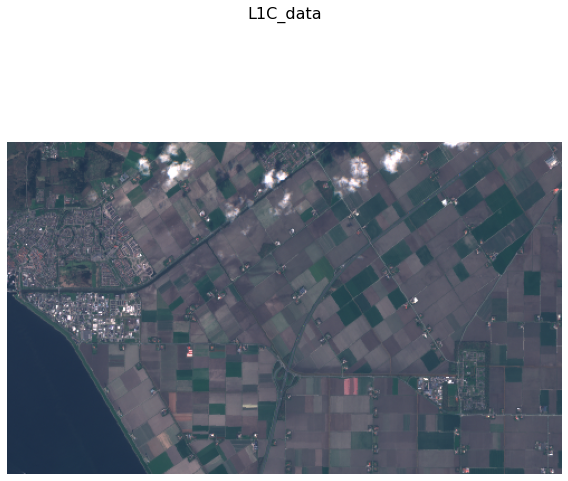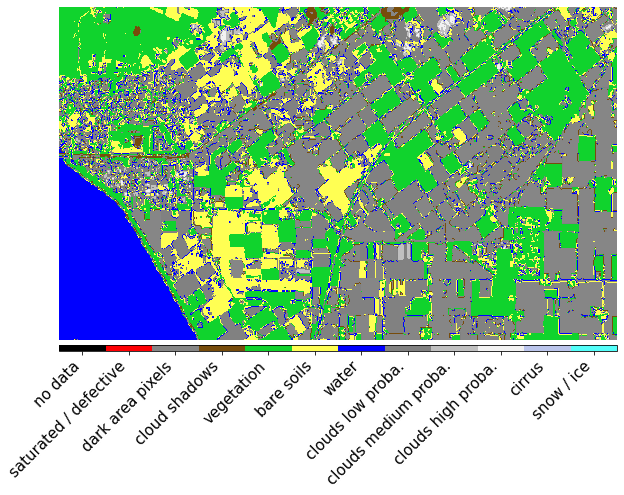:

%matplotlib inline
import matplotlib.pyplot as plt
import datetime
import numpy as np
from aenum import MultiValueEnum
from matplotlib.colors import ListedColormap, BoundaryNorm

from sentinelhub import BBox, CRS, DataCollection, MimeType, SentinelHubRequest, SHConfig



## Prerequisites

### Sentinel Hub account

In order to use Sentinel Hub services you will need a Sentinel Hub account. If you do not have one yet, you can create a free trial account at Sentinel Hub webpage. If you are a researcher you can even apply for a free non-commercial account at ESA OSEO page.

### Credentials

Please follow the instructions at configuration instructions to configure your sentinelhub installation. For Processing API request you need to obtain and set your oauth client id and secret.

Note:

Instead of providing credentials here we could also configure them beforehand according to configuration instructions.

:

# In case you put the credentials into the configuration file you can leave this unchanged

CLIENT_ID = ''
CLIENT_SECRET = ''

:

config = SHConfig()

if CLIENT_ID and CLIENT_SECRET:
config.sh_client_id = CLIENT_ID
config.sh_client_secret = CLIENT_SECRET

if config.sh_client_id == '' or config.sh_client_secret == '' or config.instance_id == '':
print("Warning! To use Sentinel Hub services, please provide the credentials (client ID and client secret).")


## Request different types of layers and data collections to an eopatch

In this workflow example, the input task requests S2 L1C bands at 20m resolution, and other eotasks add NDVI features, digital elevation model DEM, S2 L2A bands.

### General parameters

:

# region of interest
roi_bbox = BBox(bbox=[5.60, 52.68, 5.75, 52.63], crs=CRS.WGS84)

time_interval = ('2018-04-01', '2018-05-01')

# maximal cloud coverage (based on Sentinel-2 provided tile metadata)
maxcc = .8

# resolution of the request (in metres)
resolution = 20

# time difference parameter (minimum allowed time difference; if two observations are closer than this, they will be mosaicked into one observation)
time_difference = datetime.timedelta(hours=2)


#### Task for Sentinel-2 L1C data

The input_task will download all 13 Sentinel-2 bands, together with dataMask. For all possible bands that can be downloaded from Sentinel-2 data, please see Sentinel Hub documentation.

:

input_task = SentinelHubInputTask(
data_collection=DataCollection.SENTINEL2_L1C,
bands=['B01','B02','B03','B04','B05','B06','B07','B08','B8A','B09','B10','B11','B12'],
bands_feature=(FeatureType.DATA, 'L1C_data'),
resolution=resolution,
maxcc=maxcc,
time_difference=time_difference,
config=config,
)


#### Task for retrieving NDVI index

Disclaimer:

As we already have bands B04 and B08 from the previous task, we could calculate NDVI (or any other index) locally. This task serves as an example how one can use Sentinel Hub service to run the calculation.

:

indices_evalscript = """
//VERSION=3

function setup() {
return {
output:[{
id: "indices",
bands: 2,
sampleType: SampleType.FLOAT32
}]
}
}

function evaluatePixel(sample) {
let ndvi = index(sample.B08, sample.B04);
let ndwi = index(sample.B03, sample.B08);
return {
indices: [ndvi, ndwi]
};
}
"""


Note:

Requesting float32 data (SampleType.FLOAT32) from SH service consumes twice the amount of processing units compared to requesting digital numbers. Please see processing unit documentation for details.

:

# this will add two indices: ndvi and ndwi
features=[(FeatureType.DATA, 'indices')],
evalscript=indices_evalscript,
data_collection=DataCollection.SENTINEL2_L1C,
resolution=resolution,
maxcc=maxcc,
time_difference=time_difference,
config=config,
)


#### Task for Digital Elevation Model Data

:

add_dem = SentinelHubDemTask(
data_collection=DataCollection.DEM_COPERNICUS_30,
resolution=resolution,
config=config
)


#### Task for Sentinel-2 L2A and SCL data

With SentinelHubInputTask it is possible to request both L2A and SCL data in one go, optimised for smallest processing unit costs.

:

add_l2a_and_scl = SentinelHubInputTask(
data_collection=DataCollection.SENTINEL2_L2A,
bands=['B01','B02','B03','B04','B05','B06','B07','B08','B8A','B09','B11','B12'],
bands_feature=(FeatureType.DATA, 'L2A_data'),
resolution=resolution,
maxcc=maxcc,
time_difference=time_difference,
config=config,
)


#### Task for saving the eopatch

:

save = SaveTask('io_example', overwrite_permission=2, compress_level=1)


### Run workflow

:

workflow = LinearWorkflow(input_task, add_indices, add_l2a_and_scl, add_dem, save)

result = workflow.execute({
save: {'eopatch_folder': 'eopatch'}
})


Check contents of eopatch

:

eopatch = result.eopatch()
eopatch

:

EOPatch(
data: {
L1C_data: numpy.ndarray(shape=(8, 297, 497, 13), dtype=float32)
L2A_data: numpy.ndarray(shape=(8, 297, 497, 12), dtype=float32)
indices: numpy.ndarray(shape=(8, 297, 497, 2), dtype=float32)
}
SCL: numpy.ndarray(shape=(8, 297, 497, 1), dtype=uint8)
dataMask: numpy.ndarray(shape=(8, 297, 497, 1), dtype=bool)
}
scalar: {}
label: {}
vector: {}
data_timeless: {
dem: numpy.ndarray(shape=(297, 497, 1), dtype=uint16)
}
scalar_timeless: {}
label_timeless: {}
vector_timeless: {}
meta_info: {
maxcc: 0.8
size_x: 497
size_y: 297
time_difference: datetime.timedelta(seconds=1)
time_interval: None
}
bbox: BBox(((5.6, 52.63), (5.75, 52.68)), crs=CRS('4326'))
timestamp: [datetime.datetime(2018, 4, 3, 10, 41, 5, tzinfo=tzutc()), ...], length=8
)


### Plot results

#### Sentinel-2 L1C RGB bands

:

plt.figure(figsize=(10,10))
plt.imshow(np.clip(eopatch.data['L1C_data'][..., [3,2,1]] * 2.5, 0, 1), vmin=0, vmax=1);
plt.axis(False);#### NDVI data

:

plt.figure(figsize=(10,10))
plt.imshow(eopatch.data['indices'][...,0].squeeze());
plt.axis(False);#### NDWI data

:

plt.figure(figsize=(10,10))
plt.imshow(eopatch.data['indices'][...,1].squeeze());
plt.axis(False);#### Sentinel-2 L2A RGB bands

:

plt.figure(figsize=(10,10))
plt.imshow(np.clip(eopatch.data['L2A_data'][...,[3,2,1]] * 2.5, 0, 1), vmin=0, vmax=1);
plt.axis(False);#### Sentinel-2 Scene Classification Layer (from Sen2cor)

:

class SCL(MultiValueEnum):
""" Enum class containing basic LULC types
"""
NO_DATA = 'no data', 0, '#000000'
SATURATED_DEFECTIVE = 'saturated / defective', 1, '#ff0004'
DARK_AREA_PIXELS = 'dark area pixels', 2, '#868686'
VEGETATION = 'vegetation', 4, '#10d32d'
BARE_SOILS = 'bare soils', 5, '#ffff53'
WATER = 'water', 6, '#0000ff'
CLOUDS_LOW_PROBA = 'clouds low proba.', 7, '#818181'
CLOUDS_MEDIUM_PROBA = 'clouds medium proba.', 8, '#c0c0c0'
CLOUDS_HIGH_PROBA = 'clouds high proba.', 9, '#f2f2f2'
CIRRUS = 'cirrus', 10, '#bbc5ec'
SNOW_ICE = 'snow / ice', 11, '#53fffa'

@property
def rgb(self):
return [c/255. for c in self.rgb_int]

@property
def rgb_int(self):
hex_val = self.values[1:]
return [int(hex_val[i: i + 2], 16) for i in (0, 2, 4)]

scl_bounds = [-0.5 + i for i in range(len(SCL)+1)]
scl_cmap = ListedColormap([x.rgb for x in SCL], name="scl_cmap")
scl_norm = BoundaryNorm(scl_bounds, scl_cmap.N)

:

fig, ax = plt.subplots(1,1,figsize=(10,10))
plt.axis(False)

cb = fig.colorbar(im, orientation='horizontal', pad=0.01, aspect=100)
cb.ax.tick_params(labelsize=20)
cb.set_ticks([entry.values for entry in SCL])
cb.ax.set_xticklabels([entry.values for entry in SCL], rotation=45, fontsize=15, ha='right');#### Mapzen Digital Elevation Model

:

plt.figure(figsize=(10, 10))
plt.imshow(eopatch.data_timeless['dem'].squeeze());
plt.axis(False);:

load = LoadTask('io_example')

:

new_eopatch = load.execute(eopatch_folder='eopatch')